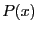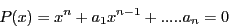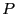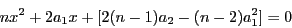Next: Implementation Up: Laguerre second method Previous: Laguerre second method   Contents

Mathematical background

Letbe an univariate polynomial of degree:Assume thathasreal roots. Then  all roots are contained in the interval whose end-point are given by the two solutions of the quadratic equation:Jean-Pierre Merlet 2012-12-20